Next: Determining algorithm performance by Up: Probabilistic Encryption Previous: Weak laws of large   Contents

## The magic of sampling

We have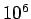envelopes. Inside each envelope is a piece of paper with 0 or 1 written on it. If we want to know exactly how many envelopes have each number, we have to open them all. Suppose we want to estimate the fraction of the envelopes of each kind, and we want the proportion to be accurate to within .05. Now we need only openenvelopes.

The situation changes dramatically if we only want to estimate the proportion with high probability. If we are willing to accept a .01 probability of an error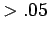, Lemma 24 implies we only need to open a randomly chosen sample of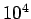envelopes9.

The special feature of problems involving squares and pseudo-squares is that sampling is possible. We saw in our discussion of the Rabin system that every number mod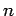has four square roots. Thus if we choose one of the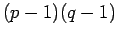numbers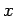not divisible10 by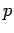or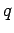and computemod n, each square has achance of being chosen. It is also important that it is possible to sample from(the set of squares and pseudo-squares) even ifare not known.

Lemma 26   There is an efficient algorithm for deciding if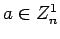.

The proof of this is difficult, involving quadratic reciprocity'' and the Jacobi symbol.'' The algorithm itself is not that complicated, and is given in the RSA paper.

Given this lemma, we can sample inby choosingat random and testing if it is in the set. If not, anotheris chosen. Since roughly half of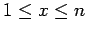is in, this won't take too long.

The different sampling possibilities we have discussed so far have all assumed that onlywas known. If we are given a single pseudo-square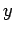, we can sample among all pseudo-squares by calculating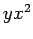forrandomly chosen.

The possibility of doing these various kinds of sampling is closely related to properties 2(a) and (c) in the paper (p. 277).

#### Footnotes

... envelopes9
Lemma 25 and Mathmatica suggest 400 envelopes are enough.
... divisible10
Even thoughare unknown, the gcd ofcan be computed.Next: Determining algorithm performance by Up: Probabilistic Encryption Previous: Weak laws of large   Contents
Translated from LaTeX by Scott Sutherland
2002-12-14